## 对称性自发破缺

### 对称性自发破缺的例子

$\mathcal{L} = \frac{1}{2}(\partial_{\mu}\phi)^2 + \frac{1}{2}\mu^2\phi^2 - \frac{\lambda}{4!}\phi^4 \tag{1}$

$H = \int d^3x [\frac{1}{2}\pi^2 + \frac{1}{2}(\nabla\phi)^2 - \frac{1}{2}\mu^2\phi^2 + \frac{\lambda}{4!}\phi^4] \tag{2}$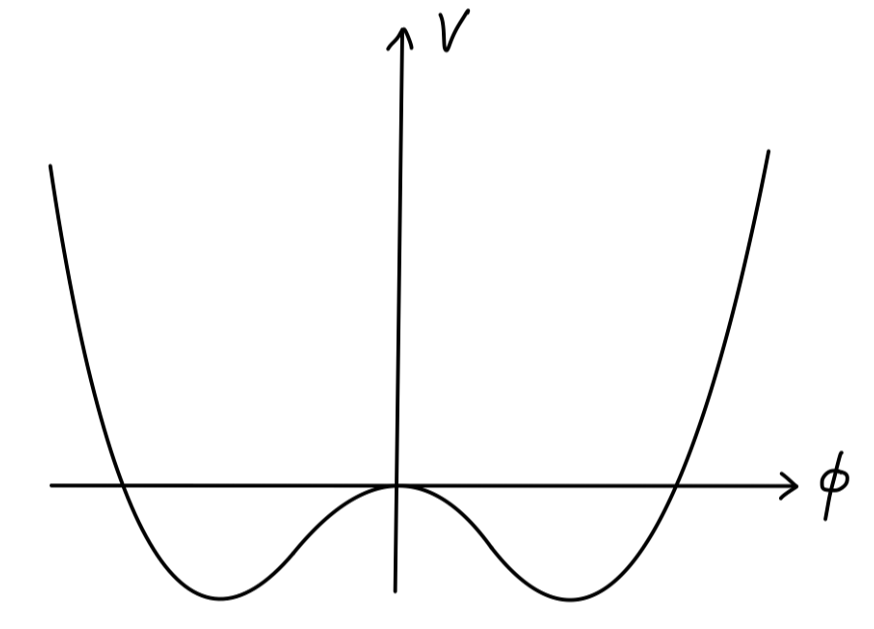$V(\phi) = - \frac{1}{2}\mu^2\phi^2 + \frac{\lambda}{4!}\phi^4\tag{3}$

$\phi_0 = \pm v= \pm \sqrt{\frac{6}{\lambda}}\mu\tag{4}$

$\phi(x) = v + \sigma(x)\tag{5}$

\begin{aligned} \mathcal{L} &= \frac{1}{2}(\partial_{\mu}\sigma)^2 - \frac{1}{2}(2\mu^2)\sigma^2 - \sqrt{\frac{\lambda}{6}}\mu\sigma^3 - \frac{\lambda}{4!}\sigma^4\\ \end{aligned}\tag{6}

$\mathcal{L} = \frac{1}{2}(\partial_{\mu}\phi^i)^2 + \frac{1}{2}\mu^2(\phi^i)^2 - \frac{\lambda}{4}[(\phi^i)^2]^2\tag{7}$

$(\phi^i)^2 = \phi^i\phi^i = \sum_{i=1}^{N}\phi_i^2$

$\phi^i \rightarrow R^{ij}\phi^j$

$V(\phi^i) = -\frac{1}{2}\mu^2(\phi^i)^2 + \frac{\lambda}{4}[(\phi^i)^2]^2 \tag{8}$

$(\phi_0^i)^2 = \frac{\mu^2}{\lambda}\tag{9}$

$\phi_0^i = (0,0,\cdots,0,v),\quad v = \frac{\mu}{\sqrt{\lambda}}\tag{10}$

$\phi^i(x) = (\pi^k(x),v+\sigma(x)),\quad k =0,\cdots,N-1 \tag{11}$

\begin{aligned} \mathcal{L} &= \frac{1}{2}(\partial_{\mu} \pi^k)^2 + \frac{1}{2}(\partial_{\mu} \sigma)^2 - \frac{1}{2}(2\mu^2)\sigma^2\\ &\quad -\sqrt{\lambda}\mu\sigma^3 - \sqrt{\lambda}\mu(\pi^k)^2\sigma - \frac{\lambda}{4}\sigma^4 - \frac{\lambda}{2}(\pi^k)^2\sigma^2 - \frac{\lambda}{4}[(\pi^k)^2]^2\\ \end{aligned}\tag{12}

## 对称性自发破缺与重整化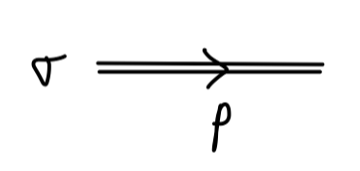$\frac{i}{p^2 - 2\mu^2}$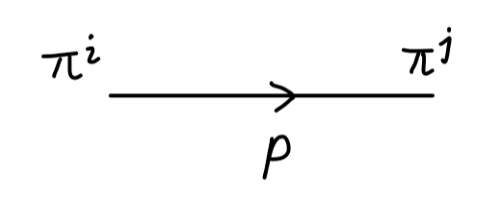$\frac{i\delta^{ij}}{p^2}$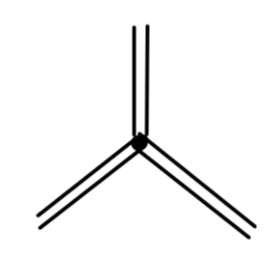$-6i\lambda v$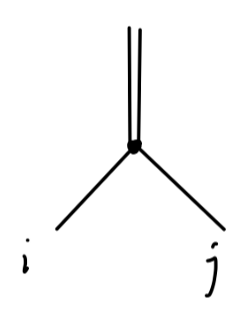$-2i\delta^{ij}\lambda v$$-6i\lambda$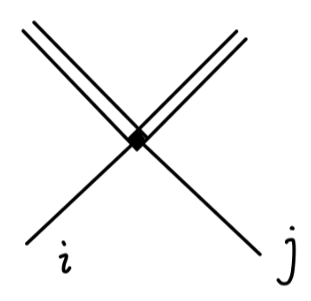$-2i\lambda\delta^{ij}$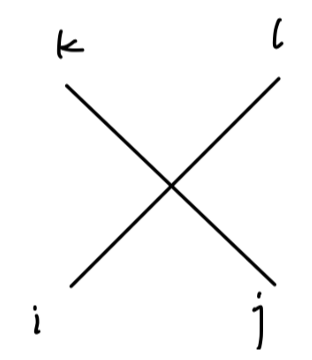$-2i\lambda[\delta^{ij}\delta^{kl} + \delta^{ik}\delta^{jl} + \delta^{il}\delta^{jk}]$

• $\sigma$-一点关联函数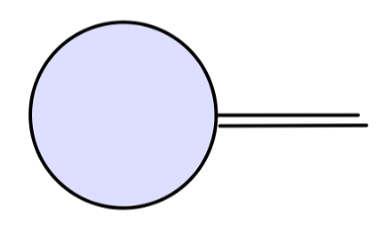• $\sigma\pi\pi$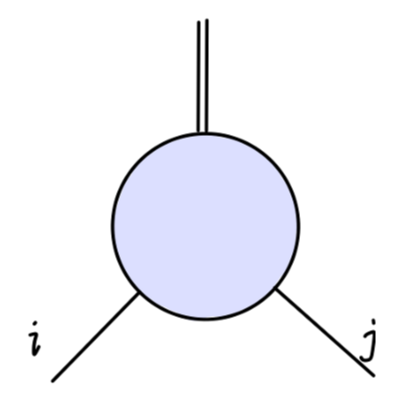• $2\sigma$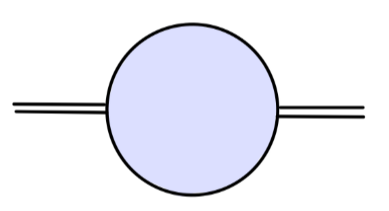• $4\sigma$ 顶点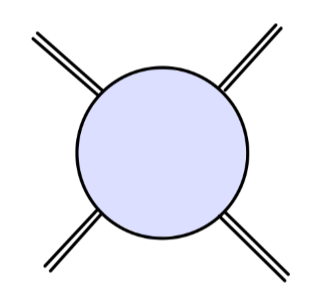• $\pi\pi$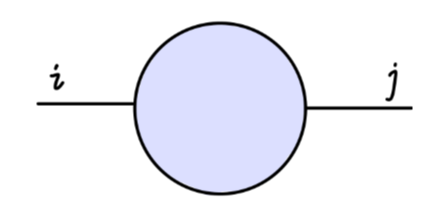• $\sigma\sigma\pi\pi$ 顶点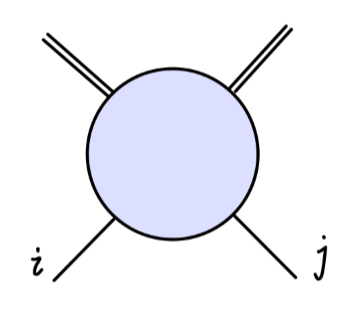• $3\sigma$ 顶点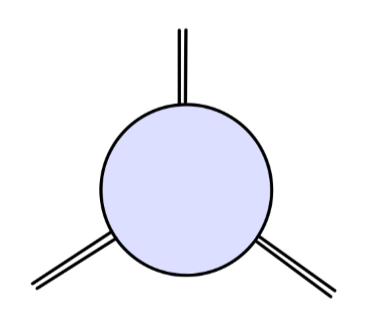• $4\pi$ 顶点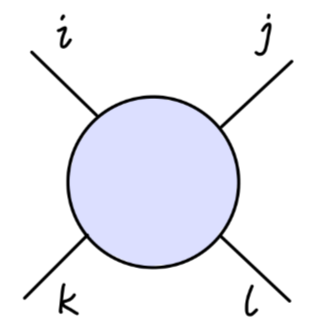\begin{aligned} \mathcal{L} &= \frac{1}{2}(\partial_{\mu}\phi^i)^2 + \frac{1}{2}\mu^2(\phi^i)^2 - \frac{\lambda}{4}[(\phi^2)^2]^2\\ &\quad + \frac{1}{2}\delta_Z(\partial_{\mu}\phi^i)^2 - \frac{1}{2}\delta_{\mu}(\phi^i)^2 - \frac{\delta_{\lambda}}{4}[(\phi^i)^2]^2 \end{aligned}

\begin{aligned} &\frac{\delta_Z}{2} (\partial_{\mu}\pi^k)^2 - \frac{1}{2}(\delta_{\mu} + \delta_{\lambda}v^2)(\pi^k)^2 + \frac{\delta_Z}{2} (\partial_{\mu}\sigma)^2 - \frac{1}{2}(\delta_{\mu} + 3\delta_{\lambda}v^2)\sigma^2\\ &- (\delta_\mu v + \delta_{\lambda}v^3)\sigma - \delta_{\lambda} v\sigma(\pi^k)^2 - \delta_{\lambda}v\sigma^3\\ &-\frac{\delta_{\lambda}}{4}[(\pi^k)^2]^2 - \frac{\delta_{\lambda}}{2}\sigma^2(\pi^k)^2 - \frac{\delta_{\lambda}}{4}\sigma^4\\ \end{aligned}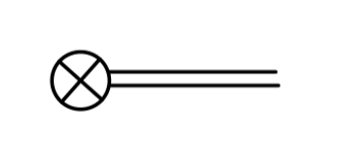$-i(\delta_{\mu}v + \delta_{\lambda}v^3)$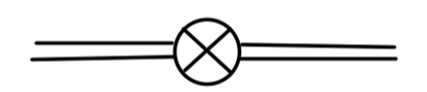$i(\delta_Z p^2 - \delta_{\mu} - 3\delta_{\lambda}v^2)$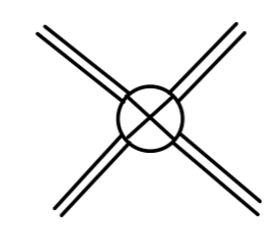$-6i\delta_{\lambda}$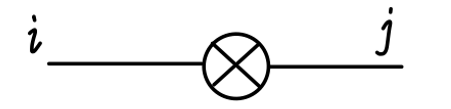$i\delta^{ij}(\delta_Z p^2 - \delta_{\mu} - \delta_{\lambda}v^2)$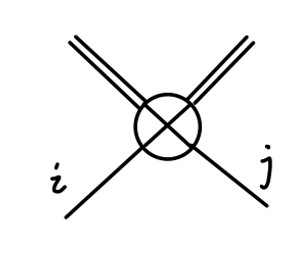$-2i\delta^{ij}\delta_{\lambda}$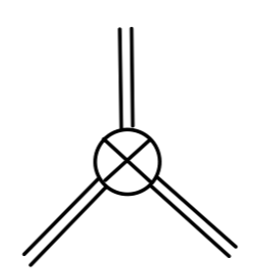$-6i\delta_{\lambda}v$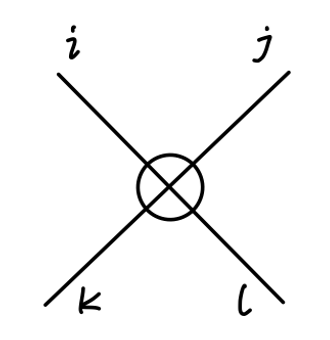$-2i\delta_{\lambda}[\delta^{ij}\delta^{kl} + \delta^{ik}\delta^{jl} + \delta^{il}\delta^{jk}]$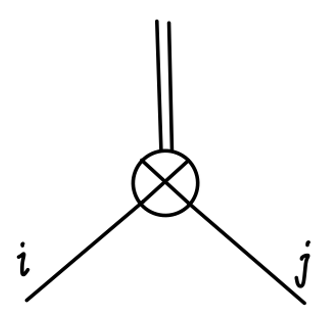$-2i\delta^{ij}\delta_{\lambda}v$

### 四点关联函数与三点关联函数

#### 重整化条件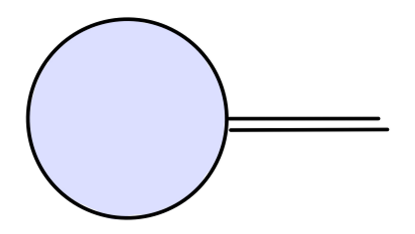$0$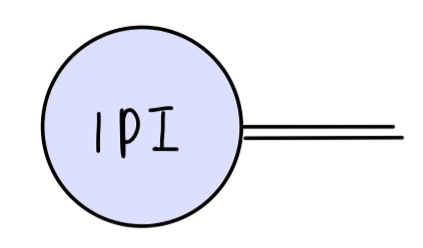$0$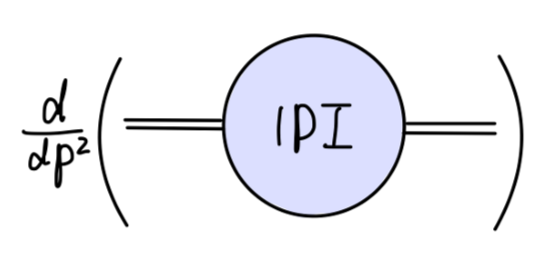$0,\quad at\ p^2 = m^2$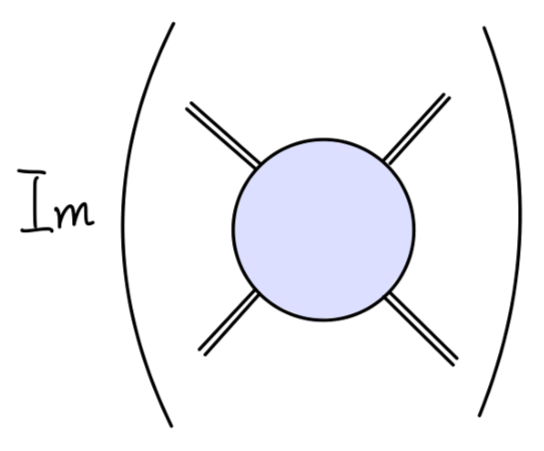$-6i\lambda \quad at\ s=4m^2,\ t=u=0$

### 重整化条件：$4\sigma$ 顶点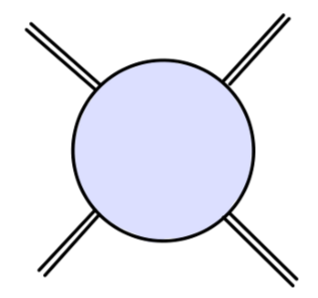$4\sigma$ 顶点的一阶圈图修正，包含 counterterms 为：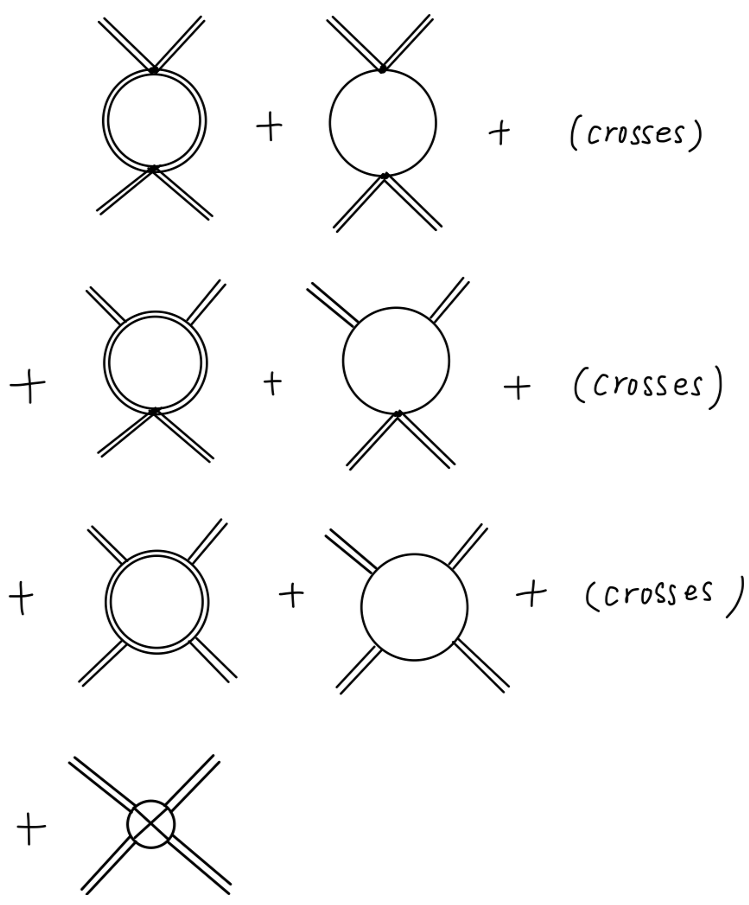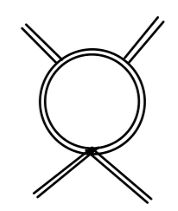$\sim \int \frac{d^4k}{(2\pi)^4}\frac{1}{k^2}\frac{1}{k^2}\frac{1}{k^2}$

$4\sigma$ 图的一阶圈图中，所有在一个圈中包含三个及其以上传播子的图（也就是二三行）都是有限值。因此我们只需要计算第一行就能确定 $\delta_{\lambda}$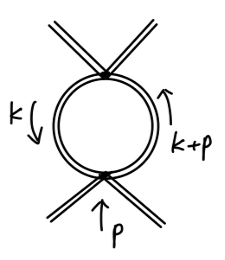\begin{aligned} &\frac{1}{2}\cdot (-6i\lambda)^2\cdot \int \frac{d^dk}{(2\pi)^d} \frac{i}{k^2-2\mu^2}\frac{i}{(k+p)^2-2\mu^2}\\ =& 18\lambda^2 \int_{0}^{1}dx \int \frac{d^dk}{(2\pi)^d} \frac{1}{[k^2-\Delta]^2}\\ =&18\lambda^2 \int_{0}^{1}dx \frac{i}{(4\pi)^{d/2}}\Gamma(2-\frac{d}{2})(\frac{1}{\Delta})^{2-\frac{d}{2}}\\ =&18i\lambda^2\frac{\Gamma(2-\frac{d}{2})}{(4\pi)^2} + (finite\ terms) \end{aligned}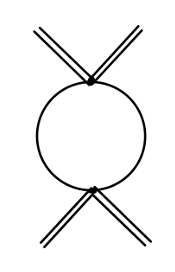$2i\lambda^2(N-1)\frac{\Gamma(2-\frac{d}{2})}{(4\pi)^2} + (finite\ terms)$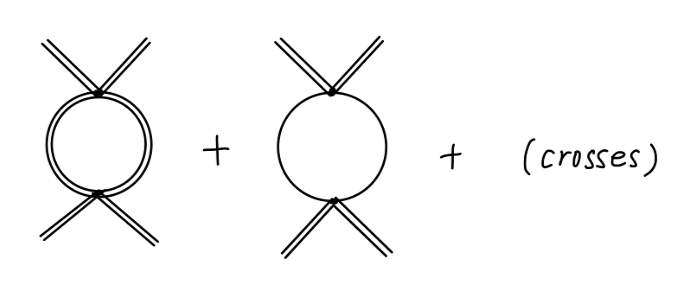$6i\lambda^2(N+8)\frac{\Gamma(2-\frac{d}{2})}{(4\pi)^2}$

$\delta_{\lambda} \sim \lambda^2(N+8)\frac{\Gamma(2-\frac{d}{2})}{(4\pi)^2}$

#### $\sigma\sigma\pi\pi$ 顶点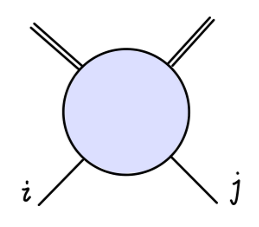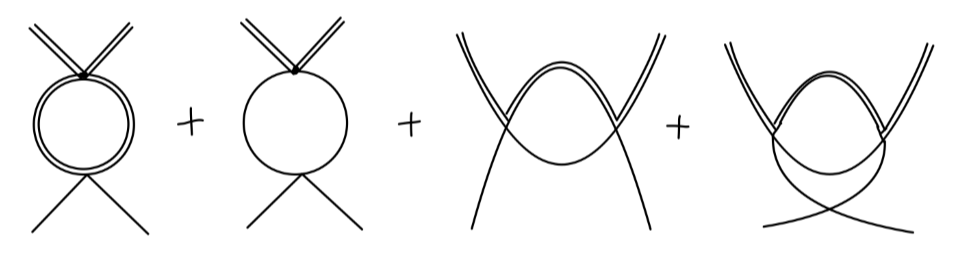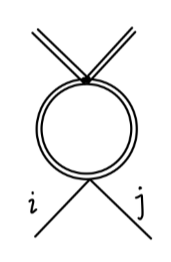$\frac{1}{2}\cdot(-6i\lambda)(-2i\lambda\delta^{ij})\cdot \frac{-i}{(4\pi)^2}\Gamma(2-\frac{d}{2}) = 6i\lambda^2\delta^{ij}\frac{\Gamma(2-\frac{d}{2})}{(4\pi)^2}$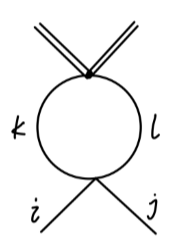\begin{aligned} &\frac{1}{2}\cdot(-2i\lambda\delta^{kl})\cdot(-2i\lambda(\delta^{ij}\delta^{kl} + \delta^{ik}\delta^{jl} + \delta^{il}\delta^{jk}))\cdot \frac{-i}{(4\pi)^2}\Gamma(2-\frac{d}{2})\\ =& 2i\lambda^2(N+1)\delta^{ij}\frac{\Gamma(2-\frac{d}{2})}{(4\pi)^2}\\ \end{aligned}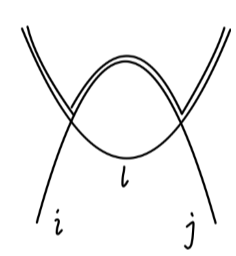\begin{aligned} &\sim (-2i\lambda\delta^{il})(-2i\lambda\delta^{jl})\cdot \frac{-i}{(4\pi)^2}\Gamma(2-\frac{d}{2})\\ &= 4i\lambda^2\delta^{ij}\frac{\Gamma(2-\frac{d}{2})}{(4\pi)^2} \end{aligned}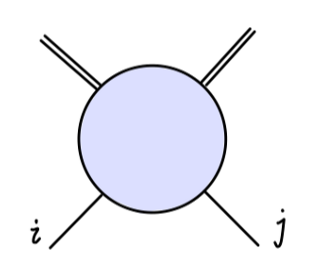$\sim 2i\lambda^2\delta^{ij}(N+8)\frac{\Gamma(2-\frac{d}{2})}{(4\pi)^2}$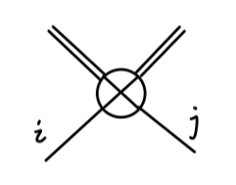\begin{aligned} &\sim -2i\delta^{ij}\delta_{\lambda}\\ &= -2i\delta^{ij}\lambda^2(N+8)\frac{\Gamma(2-\frac{d}{2})}{(4\pi)^2}\\ \end{aligned}

#### $4\pi$ 顶点

$4\pi$ 顶点的一阶修正圈图为：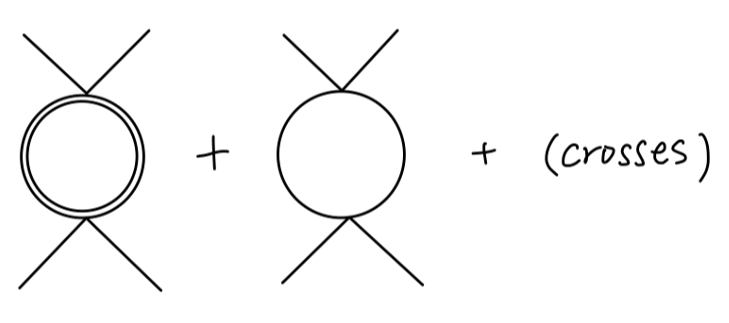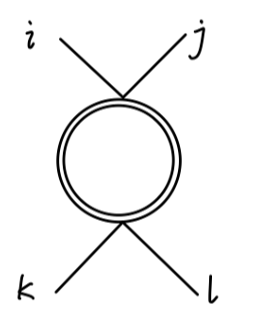\begin{aligned} &\sim \frac{1}{2}\cdot(-2i\lambda\delta^{ij})(-2i\lambda\delta^{kl})\cdot \frac{-i}{(4\pi)^2}\Gamma(2-\frac{d}{2})\\ &= 2i\lambda^2\delta^{ij}\delta^{kl}\frac{\Gamma(2-\frac{d}{2})}{(4\pi)^2}\\ \end{aligned}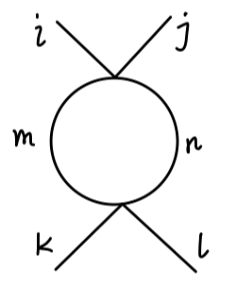\begin{aligned} &\sim \frac{1}{2}\cdot (-2i\lambda(\delta^{ij}\delta^{mn} + \delta^{im}\delta^{jn} + \delta^{in}\delta^{jm}))\\ &\qquad \cdot (-2i\lambda(\delta^{kl}\delta^{mn} + \delta^{km}\delta^{ln} + \delta^{kn}\delta^{lm}))\cdot\frac{-i}{(4\pi)^2}\Gamma(2-\frac{d}{2})\\ &= 2i\lambda^2((N+3)\delta^{ij}\delta^{kl} + 2\delta^{ik}\delta^{jl} + 2\delta^{il}\delta^{jk})\frac{\Gamma(2-\frac{d}{2})}{(4\pi)^2}\\ \end{aligned}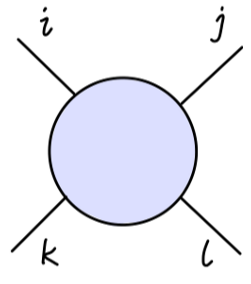$\sim 2i\lambda^2(\delta^{ij}\delta^{kl} + \delta^{ik}\delta^{jl}+ \delta^{il}\delta^{jk})(N+8)\frac{\Gamma(2-\frac{d}{2})}{(4\pi)^2}$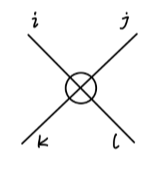\begin{aligned} &\sim -2i\delta_{\lambda}(\delta^{ij}\delta^{kl} + \delta^{ik}\delta^{jl}+ \delta^{il}\delta^{jk})\\ &= -2i\lambda^2(\delta^{ij}\delta^{kl} + \delta^{ik}\delta^{jl}+ \delta^{il}\delta^{jk})(N+8)\frac{\Gamma(2-\frac{d}{2})}{(4\pi)^2}\\ \end{aligned}

#### $3\sigma$ 顶点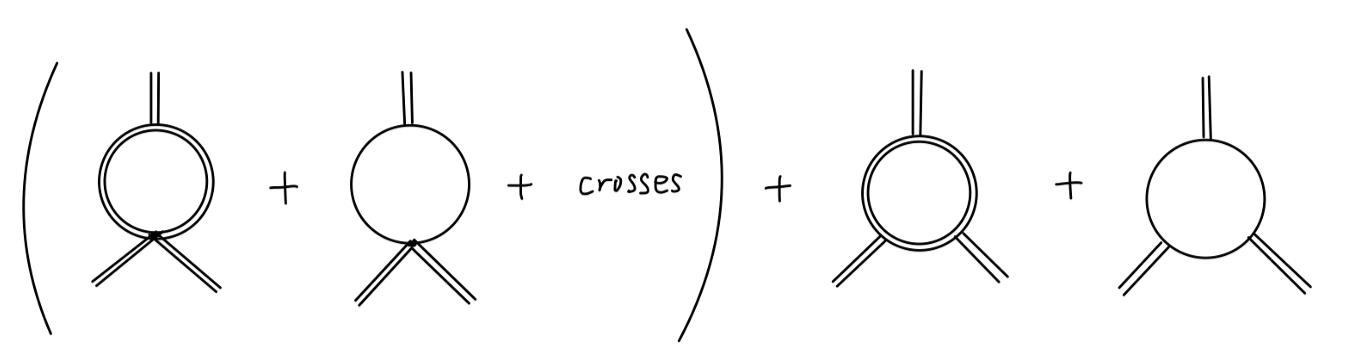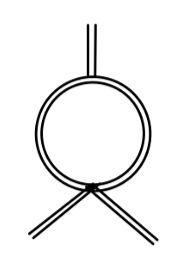\begin{aligned} &\frac{1}{2}\cdot (-6i\lambda)(-6i\lambda v) \cdot \int \frac{d^dk}{(2\pi)^d}\frac{i}{k^2-2\mu^2}\frac{i}{(k+p)^2-2\mu^2}\\ = &18i\lambda^2v \frac{\Gamma(2-\frac{d}{2})}{(4\pi)^2}\\ \end{aligned}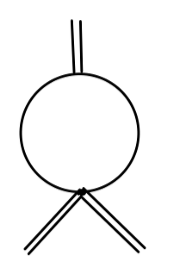$\sim 2i\lambda^2v(N-1) \frac{\Gamma(2-\frac{d}{2})}{(4\pi)^2}$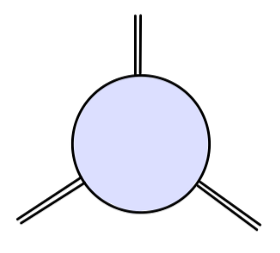$\sim 6i\lambda^2v(N+8) \frac{\Gamma(2-\frac{d}{2})}{(4\pi)^2}$

$3\sigma$ 顶点的 counterterm 为：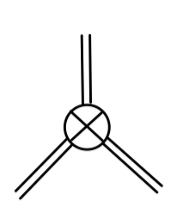\begin{aligned} &\sim -6i\delta_{\lambda}v\\ &= -6i\lambda^2v(N+8) \frac{\Gamma(2-\frac{d}{2})}{(4\pi)^2}\\ \end{aligned}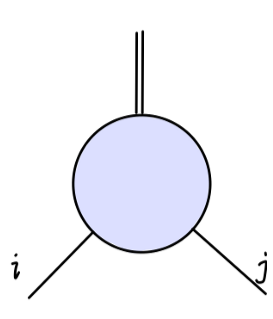### 一点关联函数与两点关联函数

#### 重整化条件：$\sigma$ 一点关联函数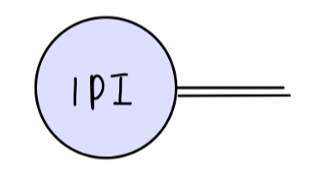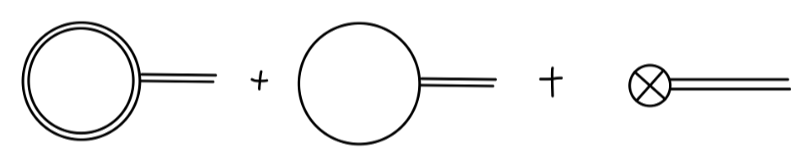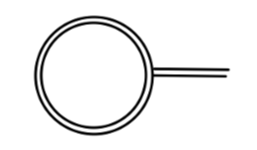\begin{aligned} &\frac{1}{2}(-6i\lambda v)\int \frac{d^dk}{(2\pi)^d}\frac{i}{k^2-2\mu^2}\\ =& -3i\lambda v \frac{\Gamma(1-\frac{d}{2})}{(4\pi)^{d/2}}(\frac{1}{2\mu^2})^{1-\frac{d}{2}}\\ \end{aligned}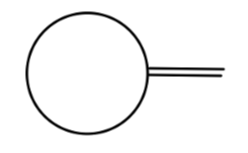\begin{aligned} &\frac{1}{2}(-2i\lambda v)\delta^{ij}\int \frac{d^dk}{(2\pi)^d}\frac{i\delta^{ij}}{k^2-\zeta^2}\\ =& -i(N-1)\lambda v \frac{\Gamma(1-\frac{d}{2})}{(4\pi)^{d/2}}(\frac{1}{\zeta^2})^{1-\frac{d}{2}}\\ \end{aligned}

$(\delta_{\mu} + v^2\delta_{\lambda}) = -\lambda\frac{\Gamma(1-\frac{d}{2})}{(4\pi)^{d/2}}(\frac{3}{(2\mu^2)^{1-d/2}} + \frac{N-1}{(\zeta^2)^{1-d/2}})\tag{13}$

#### 重整化条件：$2\sigma$ 图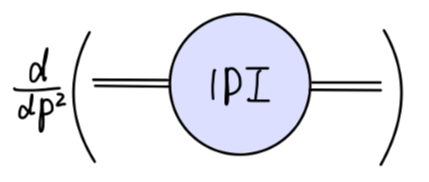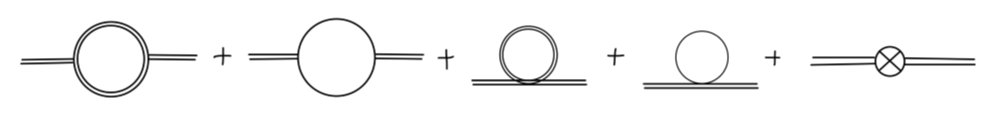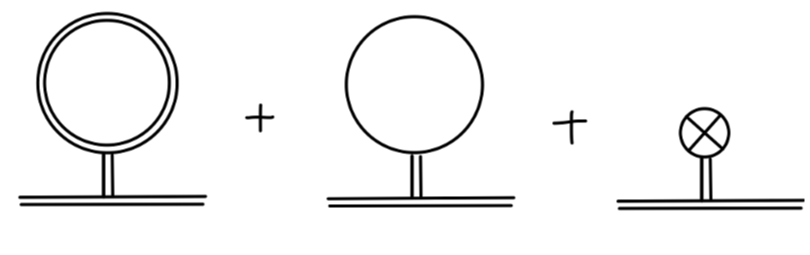$-i(2v^2\delta_{\lambda}) - i(\delta_{\mu} + v^2\delta_{\lambda}) + ip^2\delta_Z$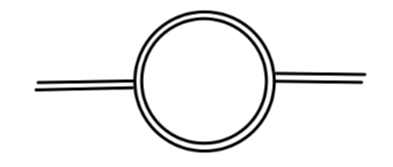$\sim 18i\lambda^2v^2\frac{\Gamma(2-\frac{d}{2})}{(4\pi)^2}$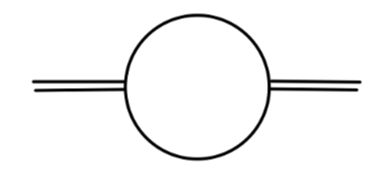$\sim 2i\lambda^2v^2(N-1)\frac{\Gamma(2-\frac{d}{2})}{(4\pi)^2}$

#### $\pi\pi$ 图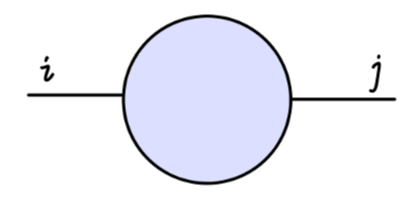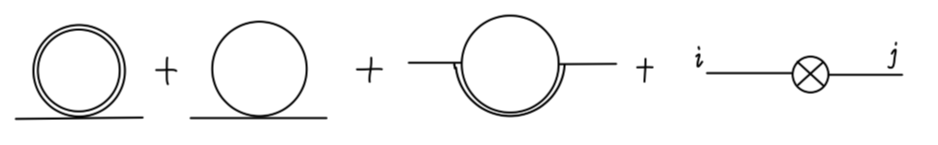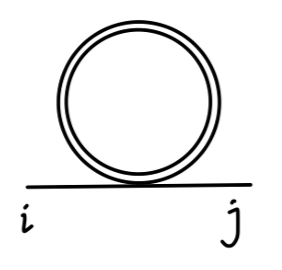\begin{aligned} &\frac{1}{2}(-2i\lambda\delta^{ij})\int \frac{d^dk}{(2\pi)^d}\frac{i}{k^2-2\mu^2}\\ =& -i\lambda\delta^{ij} \frac{\Gamma(1-\frac{d}{2})}{(4\pi)^{d/2}}(\frac{1}{2\mu^2})^{1-\frac{d}{2}}\\ \end{aligned}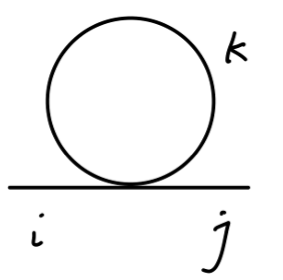\begin{aligned} &\frac{1}{2}(-2i\lambda(\delta^{ij}\delta^{kk} + \delta^{ik}\delta^{jk} + \delta^{ik}\delta^{jk}))\int \frac{d^dk}{(2\pi)^d}\frac{1}{k^2-\zeta^2}\\ =& -i\lambda(N+1)\delta^{ij} \frac{\Gamma(1-\frac{d}{2})}{(4\pi)^{d/2}}(\frac{1}{\zeta^2})^{1-\frac{d}{2}}\\ \end{aligned}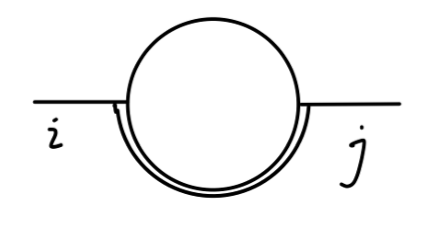\begin{aligned} &(-2i\lambda v\delta^{ik})(-2i\lambda v\delta^{kj})\int \frac{d^dk}{(2\pi)^d} \frac{i}{k^2-\zeta^2} \frac{i}{(k+p)^2 - 2\mu^2}\\ =& 4i\lambda^2 v^2 \delta^{ij}\frac{\Gamma(2-\frac{d}{2})}{(4\pi)^{d/2}} \int_{0}^{1}dx(\frac{1}{2\mu^2x + (1-x)\zeta^2 - p^2x(1-x)})^{2-\frac{d}{2}} \end{aligned}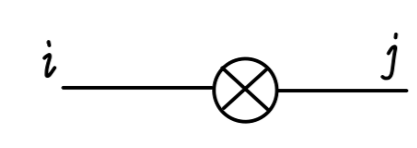\begin{aligned} &i\delta^{ij}(\delta_Z p^2 - \delta_{\mu} - \delta_{\lambda}v^2)\\ =& i\lambda \delta^{ij} \frac{\Gamma(1-\frac{d}{2})}{(4\pi)^{d/2}}(\frac{3}{(2\mu^2)^{1-d/2}} + \frac{N-1}{(\zeta^2)^{1-d/2}})\\ \end{aligned}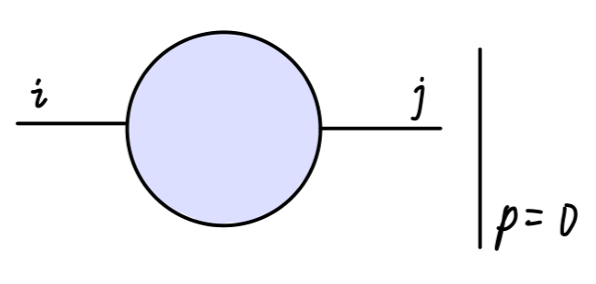\begin{aligned} (-i\lambda\delta^{ij})&\{\frac{\Gamma(1-\frac{d}{2})}{(4\pi)^{d/2}}( \frac{1}{(2\mu^2)^{1-\frac{d}{2}}} + \frac{N+1}{(\zeta^2)^{1-d/2}} )\\ &-4\lambda v^2\frac{\Gamma(2-\frac{d}{2})}{(4\pi)^{d/2}} \int_{0}^{1}dx(\frac{1}{2\mu^2x + (1-x)\zeta^2})^{2-\frac{d}{2}}\\ &-\frac{\Gamma(1-\frac{d}{2})}{(4\pi)^{d/2}}(\frac{3}{(2\mu^2)^{1-d/2}} + \frac{N-1}{(\zeta^2)^{1-d/2}})\}\\ \end{aligned}\tag{14}

$2i\lambda\delta^{ij}\frac{\Gamma(1-\frac{d}{2})}{(4\pi)^{d/2}}[\frac{1}{(\zeta^2)^{1-d/2}} - \frac{1}{(2\mu^2)^{1-d/2}}]$

$2i\lambda\delta^{ij}\frac{\Gamma(2-\frac{d}{2})}{(4\pi)^{d/2}} \frac{1}{1-d/2}[\frac{\zeta^2}{(\zeta^2)^{2-d/2}} - \frac{2\mu^2}{(2\mu^2)^{2-d/2}}]\tag{15}$

\begin{aligned} &i\lambda\delta^{ij}4\lambda v^2\frac{\Gamma(2-\frac{d}{2})}{(4\pi)^{d/2}} \frac{1}{d/2-1}\cdot \frac{(2\mu^2)^{d/2-1} - (\zeta^2)^{d/2-1}}{2\mu^2 - \zeta^2}\\ \underset{\zeta\rightarrow 0}{\longrightarrow}& i\lambda\delta^{ij}4\lambda v^2\frac{\Gamma(2-\frac{d}{2})}{(4\pi)^{d/2}} \frac{1}{d/2-1}(2\mu^2)^{d/2-2}\\ \end{aligned}\tag{16}